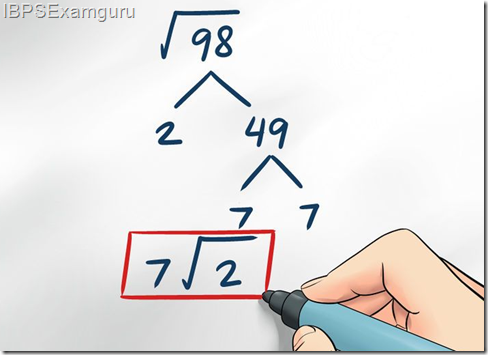Now you can Subscribe using RSS

Submit your Email

# Simplification and roots Tips & Tricks with questions

ibpsrecruitmentguru
Dear Candidates , Here Find simplification and roots Tips & Tricks with questions & Answers for Practice and Preparation purpose for all banking exams
This rule depicts the correct sequence in which the operations are to be executed, so as to
find out the value of given expression.
Here B - Bracket,
O - of,
D - Division,
M - Multiplication,
A - Addition and
S - Subtraction
Thus, in simplifying an expression, first of all the brackets must be removed, strictly in
the order (), {} and ||.
After removing the brackets, we must use the following operations strictly in the order:
(i) of (ii) Division (iii) Multiplication (iv) Addition (v) Subtraction.

### Download Here simplification and roots Tips PDFAll The Best